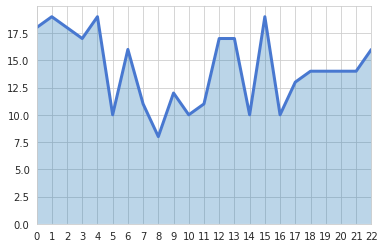You can change the figure style of a matplotlib graph using seaborn library. The example below uses 'white grid' style. This chart has been found on stack overflow, proposed by mwaskom.

``````# libraries
import numpy as np
import seaborn as sns
import matplotlib.pyplot as plt

# set the seaborn style
sns.set_style("whitegrid")

# Color palette
blue, = sns.color_palette("muted", 1)

# Create data
x = np.arange(23)
y = np.random.randint(8, 20, 23)

# Make the plot
fig, ax = plt.subplots()
ax.plot(x, y, color=blue, lw=3)
ax.fill_between(x, 0, y, alpha=.3)
ax.set(xlim=(0, len(x) - 1), ylim=(0, None), xticks=x)

# Show the graph
plt.show()``````## Contact & Edit

👋 This document is a work by Yan Holtz. You can contribute on github, send me a feedback on twitter or subscribe to the newsletter to know when new examples are published! 🔥